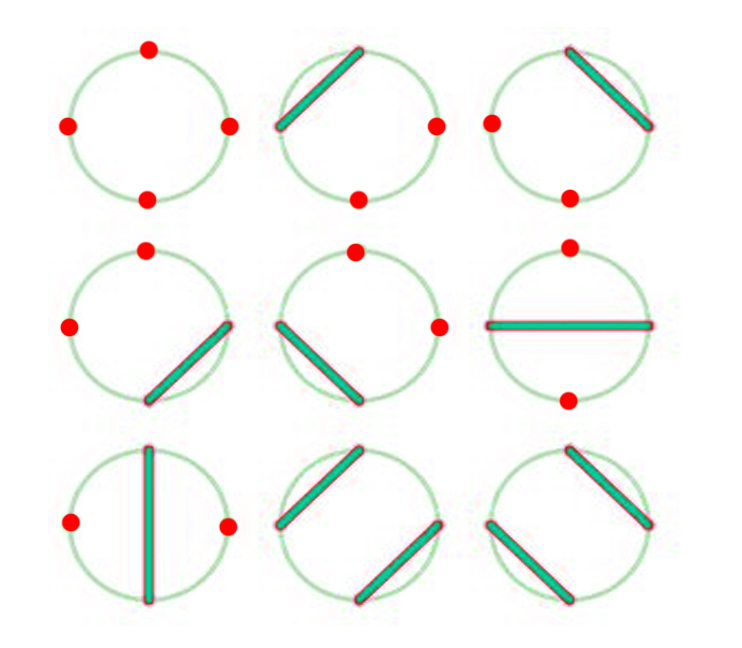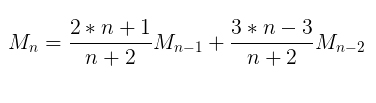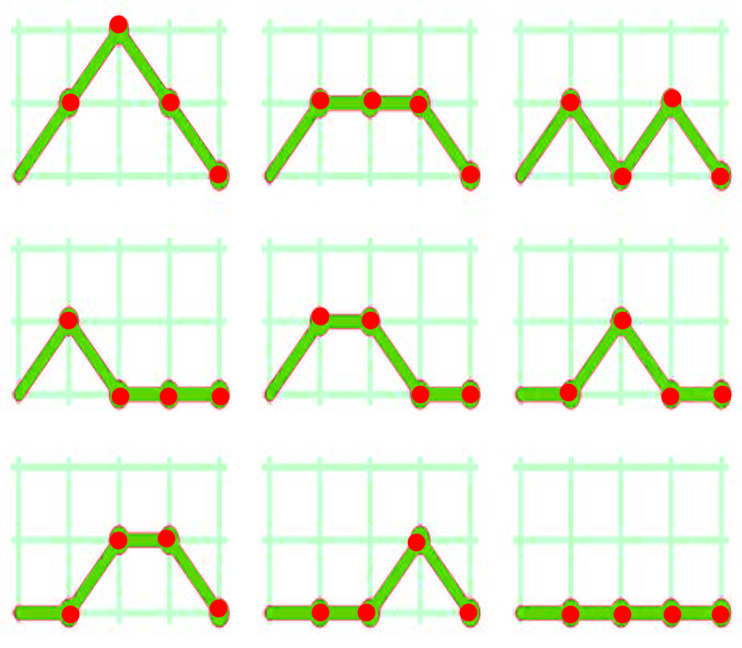Motzkin number

• Last Updated : 31 May, 2021

In mathematics, a Motzkin number for a given number n is the number of different ways of drawing non-intersecting chords between n points on a circle (not necessarily touching every point by a chord).
For example, for n = 3, M4 = 9.Recurrence relation to the Nth Motzkin Number is:Motzkin Number can be used to find:

• The number of positive integer sequences of length n – 1 in which the opening and ending elements are either 1 or 2, and the difference between any two consecutive elements is -1, 0, or 1.
• The number of routes on the upper right quadrant of a grid from coordinate (0, 0) to coordinate (n, 0) in n steps if one is allowed to move only to the right (up, down or straight) at each step but forbidden from dipping below the y = 0 axis.

For example–

The following figure shows the 9 valid Motzkin paths from (0, 0) to (4, 0).Examples:

Input : n = 4
Output : 9

Input : n = 5
Output : 21

Below is the program to find nth Motzkin Number:

C++

 // CPP Program to find Nth Motzkin Number.#include using namespace std;   // Return the nth Motzkin Number.int motzkin(int n){    // Base Case    if (n == 0 || n == 1)        return 1;       // Recursive step    return ((2 * n + 1) * motzkin(n - 1) +            (3 * n - 3) * motzkin(n - 2)) / (n + 2);}   // Driven Programint main(){    int n = 8;    cout << motzkin(n) << endl;    return 0;}

Java

 // Java Program to find Nth Motzkin Number.import java.util.*;   class Digits{    // Return the nth Motzkin Number.    public static int motzkin(int n)    {        // Base Case        if (n == 0 || n == 1)            return 1;           // Recursive step        return ((2 * n + 1) * motzkin(n - 1) +                (3 * n - 3) * motzkin(n - 2)) / (n + 2);    }           // driver code       public static void main(String[] args)    {        int n = 8;        System.out.print( motzkin(n) );    }}   // This code is contributed by rishabh_jain

Python3

 # Python3 program to find Nth Motzkin Number.   # Return the nth Motzkin Number.def motzkin(n) :           # Base Case    if (n == 0 or n == 1) :        return 1       # Recursive step    return ((2 * n + 1) * motzkin(n - 1) +            (3 * n - 3) * motzkin(n - 2)) / (n + 2)   # Driver coden = 8print( motzkin(n) )   # This code is contributed by rishabh_jain

C#

 // C# Program to find Nth Motzkin Number.using System;   class GFG {           // Return the nth Motzkin Number.    public static int motzkin(int n)    {                   // Base Case        if (n == 0 || n == 1)            return 1;           // Recursive step        return ((2 * n + 1) * motzkin(n - 1) +            (3 * n - 3) * motzkin(n - 2)) / (n + 2);    }           // driver code    public static void Main()    {        int n = 8;                   Console.WriteLine( motzkin(n) );    }}   // This code is contributed by vt_m



Javascript



Output :

323

Using Dynamic Programming :

Below is the Dynamic Programming solution of finding nth Motzkin Number :

C++

 // CPP Program to find Nth Motzkin Number.#include using namespace std;   // Return the nth Motzkin Number.int motzkin(int n){    int dp[n + 1];       // Base case    dp = dp = 1;       // Finding i-th Motzkin number.    for (int i = 2; i <= n; i++)        dp[i] = ((2 * i + 1) * dp[i - 1] +                  (3 * i - 3) * dp[i - 2]) / (i + 2);       return dp[n];}// Driven Programint main(){    int n = 8;    cout << motzkin(n) << endl;    return 0;}

Java

 // Java Program to find Nth Motzkin Number.import java.util.*;   class Digits{    // Return the nth Motzkin Number.    public static int motzkin(int n)    {        int[] dp = new int[n+1];           // Base case        dp = dp = 1;           // Finding i-th Motzkin number.        for (int i = 2; i <= n; i++)            dp[i] = ((2 * i + 1) * dp[i - 1] +                (3 * i - 3) * dp[i - 2]) / (i + 2);           return dp[n];    }           // driver code       public static void main(String[] args)    {        int n = 8;        System.out.print( motzkin(n) );    }}   // This code is contributed by rishabh_jain

Python3

 # Python3 program to find Nth Motzkin Number.   # Return the nth Motzkin Number.def motzkin(n) :           dp = [None] * (n+1)       # Base case    dp = dp = 1;       i = 2    # Finding i-th Motzkin number.    while i <= n :        dp[i] = ((2 * i + 1) * dp[i - 1] +                (3 * i - 3) * dp[i - 2]) / (i + 2);        i = i + 1    return dp[n];   # Driver coden = 8print( motzkin(n) )   # This code is contributed by rishabh_jain

C#

 // C# Program to find Nth Motzkin Number.using System;   class GFG {           // Return the nth Motzkin Number.    public static int motzkin(int n)    {        int[] dp = new int[n+1];           // Base case        dp = dp = 1;           // Finding i-th Motzkin number.        for (int i = 2; i <= n; i++)            dp[i] = ((2 * i + 1) * dp[i - 1] +             (3 * i - 3) * dp[i - 2]) / (i + 2);           return dp[n];    }           // driver code    public static void Main()    {        int n = 8;                   Console.WriteLine( motzkin(n) );    }}   // This code is contributed by vt_m

PHP



Output:

323

My Personal Notes arrow_drop_up